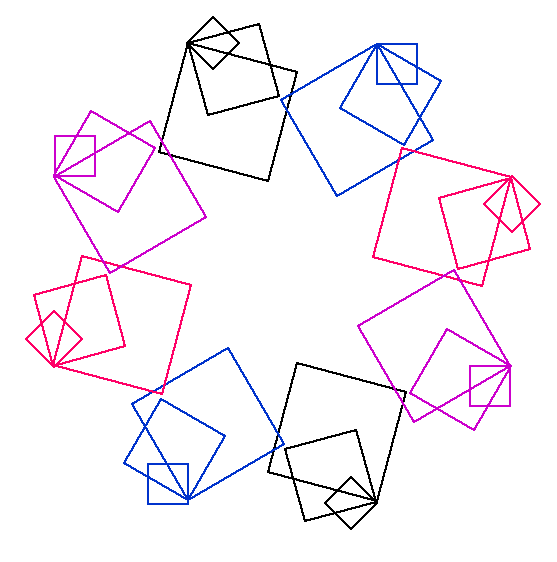You may also likeJust Opposite

A and C are the opposite vertices of a square ABCD, and have coordinates (a,b) and (c,d), respectively. What are the coordinates of the vertices B and D? What is the area of the square?Fitting In

The largest square which fits into a circle is ABCD and EFGH is a square with G and H on the line CD and E and F on the circumference of the circle. Show that AB = 5EF. Similarly the largest equilateral triangle which fits into a circle is LMN and PQR is an equilateral triangle with P and Q on the line LM and R on the circumference of the circle. Show that LM = 3PQSquare Areas

Can you work out the area of the inner square and give an explanation of how you did it?

LOGO Challenge - the Logic of LOGO

Age 11 to 16Challenge Level

Just four procedures were systematically used to produce the design below.How would you set about the task of deciding what to do? Can you use fewer procedures? How would you replicate this design?

How systematic were you in your endeavours?

You can download some excellent free LOGO software from the internet at http://fmslogo.sourceforge.net/.